• Lectures
• Code
• Notebooks
• Community

# Exchangeability and Bayesian Updating¶

## Contents¶

Co-author: Zejin Shi

## Overview¶

This lecture studies an example of learning via Bayes’ Law.

We touch on foundations of Bayesian statistical inference invented by Bruno DeFinetti [dF37].

The relevance of DeFinetti’s work for economists is presented forcefully in chapter 11 of [Kre88] by David Kreps.

The example that we study in this lecture is a key component of this lecture that augments the classic job search model of McCall [McC70] by presenting an unemployed worker with a statistical inference problem.

Here we create graphs that illustrate the role that a likelihood ratio plays in Bayes’ Law.

We’ll use such graphs to provide insights into the mechanics driving outcomes in this lecture about learning in an augmented McCall job search model.

Among other things, this lecture discusses connections between the statistical concepts of sequences of random variables that are

• independently and identically distributed
• exchangeable

Understanding the distinction between these concepts is essential for appreciating how Bayesian updating works in our example.

Below, we’ll often use

• $W$ to denote a random variable
• $w$ to denote a particular realization of a random variable $W$

In :
from numba import njit, vectorize
from math import gamma
import scipy.optimize as op
import numpy as np
import matplotlib.pyplot as plt
%matplotlib inline


### Independently and Identically Distributed¶

We begin by looking at the notion of an independently and identically distributed sequence of random variables.

An independently and identically distributed sequence is often abbreviated as IID.

Two notions are involved, independently and identically distributed.

A sequence $W_0, W_1, \ldots$ is independently distributed if the joint probability density of the sequence is the product of the densities of the components of the sequence.

The sequence $W_0, W_1, \ldots$ is independently and identically distributed if in addition the marginal density of $W_t$ is the same for all $t =0, 1, \ldots$.

For example, let $p(W_0, W_1, \ldots)$ be the joint density of the sequence and let $p(W_t)$ be the marginal density for a particular $W_t$ for all $t =0, 1, \ldots$.

Then the joint density of the sequence $W_0, W_1, \ldots$ is IID if

$$p(W_0, W_1, \ldots) = p(W_0) p(W_1) \cdots$$

so that the joint density is the product of a sequence of identical marginal densities.

### IID Means Past Observations Don’t Tell Us Anything About Future Observations¶

If a sequence is random variables is IID, past information provides no information about future realizations.

In this sense, there is nothing to learn about the future from the past.

To understand these statements, let the joint distribution of a sequence of random variables $\{W_t\}_{t=0}^T$ that is not necessarily IID, be

$$p(W_T, W_{T-1}, \ldots, W_1, W_0)$$

Using the laws of probability, we can always factor such a joint density into a product of conditional densities:

\begin{align} p(W_T, W_{T-1}, \ldots, W_1, W_0) = & p(W_T | W_{t-1}, \ldots, W_0) p(W_{T-1} | W_{T-2}, \ldots, W_0) \cdots \cr & p(W_1 | W_0) p(W_0) \end{align}

In general,

$$p(W_t | W_{t-1}, \ldots, W_0) \neq p(W_t)$$

which states that the conditional density on the left side does not equal the marginal density on the right side.

In the special IID case,

$$p(W_t | W_{t-1}, \ldots, W_0) = p(W_t)$$

and partial history $W_{t-1}, \ldots, W_0$ contains no information about the probability of $W_t$.

So in the IID case, there is nothing to learn about the densities of future random variables from past data.

In the general case, there is something go learn from past data.

We turn next to an instance of this general case in which there is something to learn from past data.

Please keep your eye out for what there is to learn from past data.

### A Setting in Which Past Observations Are Informative¶

We now turn to a setting in which there is something to learn.

Let $\{W_t\}_{t=0}^\infty$ be a sequence of nonnegative scalar random variables with a joint probability distribution constructed as follows.

There are two distinct cumulative distribution functions $F$ and $G$ — with densities $f$ and $g$ for a nonnegative scalar random variable $W$.

Before the start of time, say at time $t= -1$, “nature” once and for all selects either $f$ or $g$ — and thereafter at each time $t \geq 0$ draws a random $W$ from the selected distribution.

So the data are permanently generated as independently and identically distributed (IID) draws from either $F$ or $G$.

We could say that objectively the probability that the data are generated as draws from $F$ is either $0$ or $1$.

We now drop into this setting a decision maker who knows $F$ and $G$ and that nature picked one of them once and for all and then drew an IID sequence of draws from that distribution.

But our decision maker does not know which of the two distributions nature selected.

The decision maker summarizes his ignorance about this by picking a subjective probability $\tilde \pi$ and reasons as if nature had selected $F$ with probability $\tilde \pi \in (0,1)$ and $G$ with probability $1 - \tilde \pi$.

Thus, we assume that the decision maker

• knows both $F$ and $G$
• doesnt’t know which of these two distributions that nature has drawn
• summarizing his ignorance by acting as if or thinking that nature chose distribution $F$ with probability $\tilde \pi \in (0,1)$ and distribution $G$ with probability $1 - \tilde \pi$
• at date $t \geq 0$ has observed the partial history $w_t, w_{t-1}, \ldots, w_0$ of draws from the appropriate joint density of the partial history

But what do we mean by the appropriate joint distribution?

We’ll discuss that next and in the process describe the concept of exchangeability.

### Relationship Between IID and Exchangeable¶

Conditional on nature selecting $F$, the joint density of the sequence $W_0, W_1, \ldots$ is

$$f(W_0) f(W_1) \cdots$$

Conditional on nature selecting $G$, the joint density of the sequence $W_0, W_1, \ldots$ is

$$g(W_0) g(W_1) \cdots$$

Notice that conditional on nature having selected $F$, the sequence $W_0, W_1, \ldots$ is independently and identically distributed.

Furthermore, conditional on nature having selected $G$, the sequence $W_0, W_1, \ldots$ is also independently and identically distributed.

But what about the unconditional distribution?

The unconditional distribution of $W_0, W_1, \ldots$ is evidently

$$h(W_0, W_1, \ldots ) \equiv \tilde \pi [f(W_0) f(W_1) \cdots ] + ( 1- \tilde \pi) [g(W_0) g(W_1) \cdots ] \tag{1}$$

Under the unconditional distribution $h(W_0, W_1, \ldots )$, the sequence $W_0, W_1, \ldots$ is not independently and identically distributed.

To verify this claim, it is sufficient to notice, for example, that

$$h(w_0, w_1) = \tilde \pi f(w_0)f (w_1) + (1 - \tilde \pi) g(w_0)g(w_1) \neq (\tilde \pi f(w_0) + (1-\tilde \pi) g(w_0))( \tilde \pi f(w_1) + (1-\tilde \pi) g(w_1))$$

Thus, the conditional distribution

$$h(w_1 | w_0) \equiv \frac{h(w_0, w_1)}{(\tilde \pi f(w_0) + (1-\tilde \pi) g(w_0))} \neq ( \tilde \pi f(w_1) + (1-\tilde \pi) g(w_1))$$

This means that the realization $w_0$ contains information about $w_1$.

So there is something to learn.

But what and how?

### Exchangeability¶

While the sequence $W_0, W_1, \ldots$ is not IID, it can be verified that it is exchangeable, which means that

$$h(w_0, w_1) = h(w_1, w_0)$$

and so on.

More generally, a sequence of random variables is said to be exchangeable if the joint probability distribution for the sequence does not change when the positions in the sequence in which finitely many of the random variables appear are altered.

Equation (1) represents our instance of an exchangeable joint density over a sequence of random variables as a mixture of two IID joint densities over a sequence of random variables.

For a Bayesian statistician, the mixing parameter $\tilde \pi \in (0,1)$ has a special interpretation as a prior probability that nature selected probability distribution $F$.

DeFinetti [dF37] established a related representation of an exchangeable process created by mixing sequences of IID Bernoulli random variables with parameters $\theta$ and mixing probability $\pi(\theta)$ for a density $\pi(\theta)$ that a Bayesian statistician would interpret as a prior over the unknown Bernoulli paramter $\theta$.

### Bayes’ Law¶

We noted above that in our example model there is something to learn about about the future from past data drawn from our particular instance of a process that is exchangeable but not IID.

But how can we learn?

The answer to the about what question is about $\tilde pi$.

The answer to the how question is to use Bayes’ Law.

Another way to say use Bayes’ Law is to say compute an appropriate conditional distribution.

Let’s dive into Bayes’ Law in this context.

Let $q$ represent the distribution that nature actually draws from $w$ from and let

$$\pi = \mathbb{P}\{q = f \}$$

where we regard $\pi$ as the decision maker’s subjective probability (also called a personal probability.

Suppose that at $t \geq 0$, the decision maker has observed a history $w^t \equiv [w_t, w_{t-1}, \ldots, w_0]$.

We let

$$\pi_t = \mathbb{P}\{q = f | w^t \}$$

$$\pi_{-1} = \tilde \pi$$

The distribution of $w_{t+1}$ conditional on $w^t$ is then

$$\pi_t f + (1 - \pi_t) g .$$

Bayes’ rule for updating $\pi_{t+1}$ is

$$\pi_{t+1} = \frac{\pi_t f(w_{t+1})}{\pi_t f(w_{t+1}) + (1 - \pi_t) g(w_{t+1})} \tag{2}$$

The last expression follows from Bayes’ rule, which tells us that

$$\mathbb{P}\{q = f \,|\, W = w\} = \frac{\mathbb{P}\{W = w \,|\, q = f\}\mathbb{P}\{q = f\}} {\mathbb{P}\{W = w\}} \quad \text{and} \quad \mathbb{P}\{W = w\} = \sum_{\omega \in \{f, g\}} \mathbb{P}\{W = w \,|\, q = \omega\} \mathbb{P}\{q = \omega\}$$

### More Details about Bayesian Updating¶

Let’s stare at and rearrange Bayes’ Law as represented in equation (2) with the aim of understanding how the posterior $\pi_{t+1}$ is influenced by the prior $\pi_t$ and the likelihood ratio

$$l(w) = \frac{f(w)}{g(w)}$$

It is convenient for us to rewrite the updating rule (2) as

$$\pi_{t+1} =\frac{\pi_{t}f\left(w_{t+1}\right)}{\pi_{t}f\left(w_{t+1}\right)+\left(1-\pi_{t}\right)g\left(w_{t+1}\right)} =\frac{\pi_{t}\frac{f\left(w_{t+1}\right)}{g\left(w_{t+1}\right)}}{\pi_{t}\frac{f\left(w_{t+1}\right)}{g\left(w_{t+1}\right)}+\left(1-\pi_{t}\right)} =\frac{\pi_{t}l\left(w_{t+1}\right)}{\pi_{t}l\left(w_{t+1}\right)+\left(1-\pi_{t}\right)}$$

This implies that

$$\frac{\pi_{t+1}}{\pi_{t}}=\frac{l\left(w_{t+1}\right)}{\pi_{t}l\left(w_{t+1}\right)+\left(1-\pi_{t}\right)}\begin{cases} >1 & \text{if }l\left(w_{t+1}\right)>1\\ \leq1 & \text{if }l\left(w_{t+1}\right)\leq1 \end{cases} \tag{3}$$

Notice how the likelihood ratio and the prior interact to determine whether an observation $w_{t+1}$ leads the decision maker to increase or decrease the subjective probability he/she attaches to distribution $F$.

When the likelihood ratio $l(w_{t+1})$ exceeds one, the observation $w_{t+1}$ nudges the probability $\pi$ put on distribution $F$ upward, and when the likelihood ratio $l(w_{t+1})$ is less that one, the observation $w_{t+1}$ nudges $\pi$ downward.

Representation (3) is the foundation of the graphs that we’ll use to display the dynamics of $\{\pi_t\}_{t=0}^\infty$ that are induced by Bayes’ Law.

We’ll plot $l\left(w\right)$ as a way to enlighten us about how learning – i.e., Bayesian updating of the probability $\pi$ that nature has chosen distribution $f$ – works.

To create the Python infrastructure to do our work for us, we construct a wrapper function that displays informative graphs given parameters of $f$ and $g$.

In :
@vectorize
def p(x, a, b):
"The general beta distribution function."
r = gamma(a + b) / (gamma(a) * gamma(b))
return r * x ** (a-1) * (1 - x) ** (b-1)

def learning_example(F_a=1, F_b=1, G_a=3, G_b=1.2):
"""
A wrapper function that displays the updating rule of belief π,
given the parameters which specify F and G distributions.
"""

f = njit(lambda x: p(x, F_a, F_b))
g = njit(lambda x: p(x, G_a, G_b))

# l(w) = f(w) / g(w)
l = lambda w: f(w) / g(w)
# objective function for solving l(w) = 1
obj = lambda w: l(w) - 1

x_grid = np.linspace(0, 1, 100)
π_grid = np.linspace(1e-3, 1-1e-3, 100)

w_max = 1
w_grid = np.linspace(1e-12, w_max-1e-12, 100)

# the mode of beta distribution
# use this to divide w into two intervals for root finding
G_mode = (G_a - 1) / (G_a + G_b - 2)
roots = np.empty(2)
roots = op.root_scalar(obj, bracket=[1e-10, G_mode]).root
roots = op.root_scalar(obj, bracket=[G_mode, 1-1e-10]).root

fig, (ax1, ax2, ax3) = plt.subplots(1, 3, figsize=(18, 5))

ax1.plot(l(w_grid), w_grid, label='$l$', lw=2)
ax1.vlines(1., 0., 1., linestyle="--")
ax1.hlines(roots, 0., 2., linestyle="--")
ax1.set_xlim([0., 2.])
ax1.legend(loc=4)
ax1.set(xlabel='$l(w)=f(w)/g(w)$', ylabel='$w$')

ax2.plot(f(x_grid), x_grid, label='$f$', lw=2)
ax2.plot(g(x_grid), x_grid, label='$g$', lw=2)
ax2.vlines(1., 0., 1., linestyle="--")
ax2.hlines(roots, 0., 2., linestyle="--")
ax2.legend(loc=4)
ax2.set(xlabel='$f(w), g(w)$', ylabel='$w$')

ax2.text((f(0) + f(roots)) / 4, roots / 2, f"{area1: .3g}")
ax2.fill_between([0, 1], 0, roots, color='blue', alpha=0.15)
ax2.text(np.mean(g(roots)) / 2, np.mean(roots), f"{area2: .3g}")
w_roots = np.linspace(roots, roots, 20)
ax2.fill_betweenx(w_roots, 0, g(w_roots), color='orange', alpha=0.15)
ax2.text((f(roots) + f(1)) / 4, (roots + 1) / 2, f"{area3: .3g}")
ax2.fill_between([0, 1], roots, 1, color='blue', alpha=0.15)

W = np.arange(0.01, 0.99, 0.08)
Π = np.arange(0.01, 0.99, 0.08)

ΔW = np.zeros((len(W), len(Π)))
ΔΠ = np.empty((len(W), len(Π)))
for i, w in enumerate(W):
for j, π in enumerate(Π):
lw = l(w)
ΔΠ[i, j] = π * (lw / (π * lw + 1 - π) - 1)

q = ax3.quiver(Π, W, ΔΠ, ΔW, scale=2, color='r', alpha=0.8)

ax3.fill_between(π_grid, 0, roots, color='blue', alpha=0.15)
ax3.fill_between(π_grid, roots, roots, color='green', alpha=0.15)
ax3.fill_between(π_grid, roots, w_max, color='blue', alpha=0.15)
ax3.hlines(roots, 0., 1., linestyle="--")
ax3.set(xlabel='$\pi$', ylabel='$w$')
ax3.grid()

plt.show()


Now we’ll create a group of graphs designed to illustrate the dynamics induced by Bayes’ Law.

We’ll begin with the default values of various objects, then change them in a subsequent example.

In :
learning_example()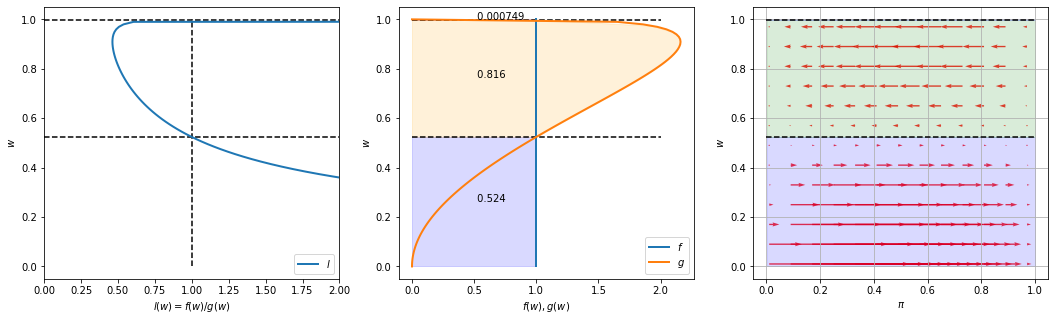Please look at the three graphs above created for an instance in which $f$ is a uniform distribution on $[0,1]$ (i.e., a Beta distribution with parameters $F_a=1, F_b=1$, while $g$ is a Beta distribution with the default parameter values $G_a=3, G_b=1.2$.

The graph in the left plots the likehood ratio $l(w)$ on the coordinate axis against $w$ on the coordinate axis.

The middle graph plots both $f(w)$ and $g(w)$ against $w$, with the horizontal dotted lines showing values of $w$ at which the likelihood ratio equals $1$.

The graph on the right side plots arrows to the right that show when Bayes’ Law makes $\pi$ increase and arrows to the left that show when Bayes’ Law make $\pi$ decrease.

Notice how the length of the arrows, which show the magnitude of the force from Bayes’ Law impelling $\pi$ to change, depend on both the prior probability $\pi$ on the ordinate axis and the evidence in the form of the current draw of $w$ on the coordinate axis.

The fractions in the colored areas of the middle graphs are probabilities under $F$ and $G$, respectively, that realizations of $w$ fall into the interval that updates the belief $\pi$ in a correct direction (i.e., toward $0$ when $G$ is the true distribution, and towards $1$ when $F$ is the true distribution).

For example, in the above example, under true distribution $F$, $\pi$ will be updated toward $0$ if $w$ falls into the interval $[0.524, 0.999]$, which occurs with probability $1 - .524 = .476$ under $F$. But this would occur with probability $0.816$ if $G$ were the true distribution. The fraction $0.816$ in the orange region is the integral of $g(w)$ over this interval.

Next we use our code to create graphs for another instance of our model.

We keep $F$ the same as in the preceding instance, namely a uniform distribution, but now assume that $G$ is a Beta distribution with parameters $G_a=2, G_b=1.6$.

In :
learning_example(G_a=2, G_b=1.6)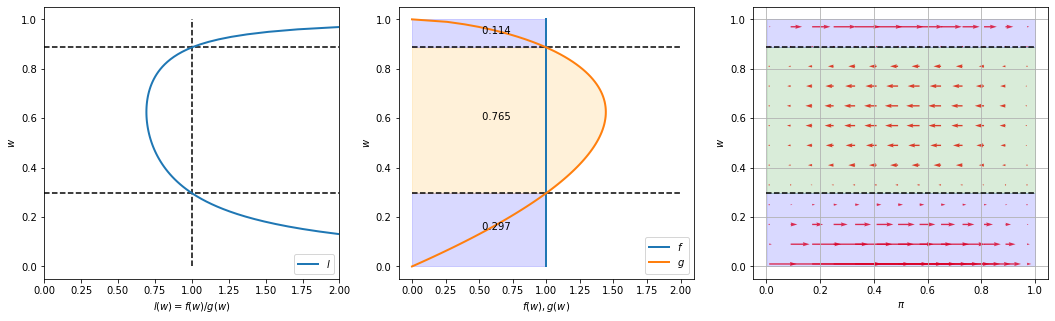Notice how the likelihood ratio, the middle graph, and the arrows compare with the previous instance of our example.

## Appendix¶

### Sample Paths of $\pi_t$¶

Now we’ll have some fun by plotting multiple realizations of sample paths of $\pi_t$ under two possible assumptions about nature’s choice of distribution:

• that nature permanently draws from $F$
• that nature permanently draws from $G$

Outcomes depend on a peculiar property of likelihood ratio processes that are discussed in this lecture

To do this, we create some Python code.

In :
def function_factory(F_a=1, F_b=1, G_a=3, G_b=1.2):

# define f and g
f = njit(lambda x: p(x, F_a, F_b))
g = njit(lambda x: p(x, G_a, G_b))

@njit
def update(a, b, π):
"Update π by drawing from beta distribution with parameters a and b"

# Draw
w = np.random.beta(a, b)

# Update belief
π = 1 / (1 + ((1 - π) * g(w)) / (π * f(w)))

return π

@njit
def simulate_path(a, b, T=50):
"Simulates a path of beliefs π with length T"

π = np.empty(T+1)

# initial condition
π = 0.5

for t in range(1, T+1):
π[t] = update(a, b, π[t-1])

return π

def simulate(a=1, b=1, T=50, N=200, display=True):
"Simulates N paths of beliefs π with length T"

π_paths = np.empty((N, T+1))
if display:
fig = plt.figure()

for i in range(N):
π_paths[i] = simulate_path(a=a, b=b, T=T)
if display:
plt.plot(range(T+1), π_paths[i], color='b', lw=0.8, alpha=0.5)

if display:
plt.show()

return π_paths

return simulate

In :
simulate = function_factory()


We begin by generating $N$ simulated $\{\pi_t\}$ paths with $T$ periods when the sequence is truly IID draws from $F$. We set the initial prior $\pi_{-1} = .5$.

In :
T = 50

In :
# when nature selects F
π_paths_F = simulate(a=1, b=1, T=T, N=1000)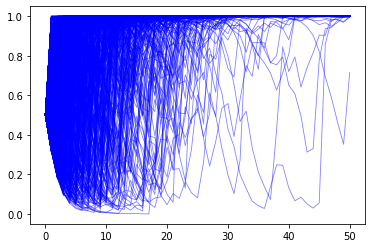In the above graph we observe that for most paths $\pi_t \rightarrow 1$. So Bayes’ Law evidently eventually discovers the truth for most of our paths.

Next, we generate paths with $T$ periods when the sequence is truly IID draws from $G$. Again, we set the initial prior $\pi_{-1} = .5$.

In :
# when nature selects G
π_paths_G = simulate(a=3, b=1.2, T=T, N=1000)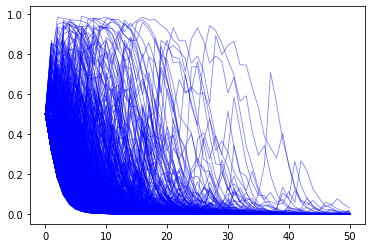In the above graph we observe that now most paths $\pi_t \rightarrow 0$.

### Rates of convergence¶

We study rates of convergence of $\pi_t$ to $1$ when nature generates the data as IID draws from $F$ and of $\pi_t$ to $0$ when nature generates the data as IID draws from $G$.

We do this by averaging across simulated paths of $\{\pi_t\}_{t=0}^T$.

Using $N$ simulated $\pi_t$ paths, we compute $1 - \sum_{i=1}^{N}\pi_{i,t}$ at each $t$ when the data are generated as draws from $F$ and compute $\sum_{i=1}^{N}\pi_{i,t}$ when the data are generated as draws from $G$.

In :
plt.plot(range(T+1), 1 - np.mean(π_paths_F, 0), label='F generates')
plt.plot(range(T+1), np.mean(π_paths_G, 0), label='G generates')
plt.legend()
plt.title("convergence");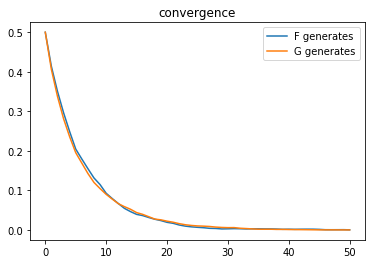From the above graph, rates of convergence appear not to depend on whether $F$ or $G$ generates the data.

### Another Graph of Population Dynamics of $\pi_t$¶

More insights about the dynamics of $\{\pi_t\}$ can be gleaned by computing the following conditional expectations of $\frac{\pi_{t+1}}{\pi_{t}}$ as functions of $\pi_t$ via integration with respect to the pertinent probability distribution:

\begin{aligned} E\left[\frac{\pi_{t+1}}{\pi_{t}}\biggm|q=\omega, \pi_{t}\right] &=E\left[\frac{l\left(w_{t+1}\right)}{\pi_{t}l\left(w_{t+1}\right)+\left(1-\pi_{t}\right)}\biggm|q=\omega, \pi_{t}\right], \\ &=\int_{0}^{1}\frac{l\left(w_{t+1}\right)}{\pi_{t}l\left(w_{t+1}\right)+\left(1-\pi_{t}\right)}\omega\left(w_{t+1}\right)dw_{t+1} \end{aligned}

where $\omega=f,g$.

The following code approximates the integral above:

In :
def expected_ratio(F_a=1, F_b=1, G_a=3, G_b=1.2):

# define f and g
f = njit(lambda x: p(x, F_a, F_b))
g = njit(lambda x: p(x, G_a, G_b))

l = lambda w: f(w) / g(w)
integrand_f = lambda w, π: f(w) * l(w) / (π * l(w) + 1 - π)
integrand_g = lambda w, π: g(w) * l(w) / (π * l(w) + 1 - π)

π_grid = np.linspace(0.02, 0.98, 100)

expected_rario = np.empty(len(π_grid))
for q, inte in zip(["f", "g"], [integrand_f, integrand_g]):
for i, π in enumerate(π_grid):
plt.plot(π_grid, expected_rario, label=f"{q} generates")

plt.hlines(1, 0, 1, linestyle="--")
plt.xlabel("$π_t$")
plt.ylabel("$E[\pi_{t+1}/\pi_t]$")
plt.legend()

plt.show()


First, consider the case where $F_a=F_b=1$ and $G_a=3, G_b=1.2$.

In :
expected_ratio()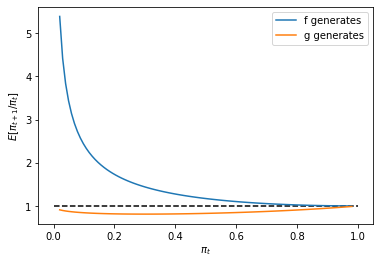The above graphs shows that when $F$ generates the data, $\pi_t$ on average always heads north, while when $G$ generates the data, $\pi_t$ heads south.

Next, we’ll look at a degenerate case in whcih $f$ and $g$ are identical beta distributions, and $F_a=G_a=3, F_b=G_b=1.2$.

In a sense, here there is nothing to learn.

In :
expected_ratio(F_a=3, F_b=1.2)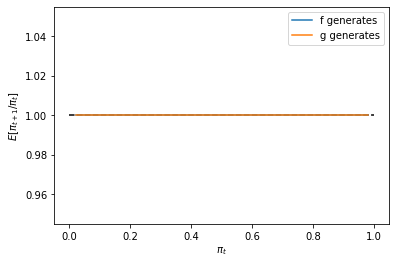The above graph says that $\pi_t$ is inert and would remain at its initial value.

Finally, let’s look at a case in which $f$ and $g$ are neither very different nor identical, in particular one in which $F_a=2, F_b=1$ and $G_a=3, G_b=1.2$.

In :
expected_ratio(F_a=2, F_b=1, G_a=3, G_b=1.2)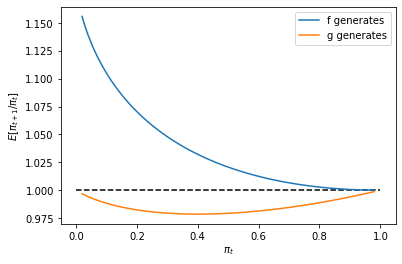• Share page